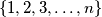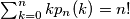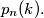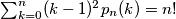### IMO Shortlist 1987 problem 16

Kvaliteta:
Avg: 0.0
Težina:
Avg: 6.0
Let$p_n(k)$ be the number of permutations of the set$\{1,2,3,\ldots,n\}$ which have exactly$k$ fixed points. Prove that$\sum_{k=0}^nk p_n(k)=n!$.(IMO Problem 1)

Original formulation

Let$S$ be a set of$n$ elements. We denote the number of all permutations of$S$ that have exactly$k$ fixed points by$p_n(k).$ Prove:

(a)$\sum_{k=0}^{n} kp_n(k)=n! \ ;$

(b)$\sum_{k=0}^{n} (k-1)^2 p_n(k) =n!$

Proposed by Germany, FR
Source: Međunarodna matematička olimpijada, shortlist 1987